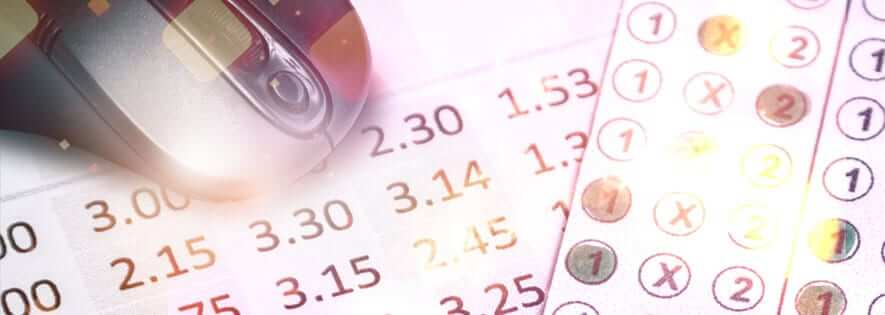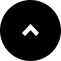# Guide to Betting Odds

Knowing what betting odds represent is essential for anyone new to the world of gambling. How do odds work, what information do they contain and what will the return be if a bet wins?

Knowing what betting odds represent is essential for anyone new to the world of gambling. How do odds work, what information do they contain and what will the return be if a bet wins?

### Betting Odds = Stake vs. Net Return

Essentially, betting odds show the relationship between:

• The size of your chosen stake, and
• The relative proportion of money that will be returned to you (in addition to your stake) should your bet win

### Betting Odds = Implied Probabilities of Success

Odds also carry information about one other thing:

• The implied probability of the outcome in question (i.e. the rough percentage chance of it occurring)

But no-one has a crystal ball and no-one can foresee the future. Opinion and circumstances change all the time and, as a result, odds fluctuate from the opening of the market to the close of the event.

These variables are why it is crucial to understand that odds can only ever represent implied probabilities. In other words, odds offer a guide to the ‘rough’ or ‘implied’ chances (based on public opinion) of a particular outcome taking place – They can never, ever be precise.

### What Are The Chances?

• Evens (exactly 50% chance)
• Below Evens (below 50% chance)
• Above Evens (above 50% chance)

Evens (50%)

A flip of a coin: there are only two possible outcomes in a situation such as this and each has an equal or ‘even’ chance of happening; 50% heads or 50% tails. (Simply, 100% divided by two).

With evens bets, the net reward on offer is always the same size as the amount staked.

N.B. In American parlance, this bet is referred to as being directly on the ‘Moneyline’, i.e. exactly 50%.

Below Evens

Roll of dice: the chance of seeing any nominated number is one-in-six, which equates to a 16.67% chance. (Simply, 100% divided by six).

With below evens bets, the net reward on offer is always higher than the amount staked.

N.B. This is a positive ‘Moneyline’ bet – chance of success is below 50%.

Above Evens

Football team avoiding defeat: two of the three possible outcomes of a match (i.e. winning or drawing the game – the ‘double chance’ market) is 66.67%. (Simply, 100% divided by three, multiplied by two).

With above evens bets, the net reward on offer is always less than the amount staked.

N.B. This is a negative ‘Moneyline’ bet – chance of success is above 50%.

PROBABILITIES RULE OF THUMB:

The higher the probability of success, the lower the reward, and vice versa…

### Odds Formats

Geographically concentrated, there are three main odds formats used:

• Fractional odds (old-school British)
• “Moneyline” odds (North American)
• Decimal odds (European)

Decimal odds are the most widespread online, although most bookmaker platforms provide a choice of formats depending on your location and preference.

### Fractional Odds

Fractional odds are still in use by bookmakers in the UK and Ireland, although decimal odds are fast replacing them.

Fractional odds quote the proportional net return that will be paid out to the bettor should he win, relative to his stake.

• The notation for an ‘evens’ or ‘even money’ 50% probability bet is 1/1, or one-to-one (one returned for every one staked). This is sometimes written as Ev/Ev or Evens/Evens.
• For our ‘below evens’ example (16.67% probability), fractional odds would be 5/1, or five-to-one (five returned for every one staked).
• For our ‘above evens’ example (66.67% probability), fractional odds would be 1/2, or one-to-two (one returned for every two staked).

The formula to convert implied probabilities into fractional odds is:

(100/implied probability) – 1

E.g. (100/16.67) – 1 = 5 (-to-one)

To reverse fractional odds into their implied probabilities:

1 / (odds + 1)

E.g. 1 divided by (5 + 1) = 16.67%

### Moneyline Odds

Moneyline odds are almost exclusively used by North American bookmakers and are designed to show the stake required to win 100 units or, the money which will be won from a 100 unit wager.

• The Moneyline notation for an ‘evens’ or ‘even money’ 50% probability bet is +100, or one-hundred returned for every one-hundred staked.
• For our ‘below evens’ example (16.67% probability), Moneyline odds would be +500, or five-hundred returned for every one-hundred staked.
• For our ‘above evens’ example (66.67% probability), fractional odds would be -200, or two-hundred stake required to win one-hundred.

The formulas to convert implied probabilities into Moneyline odds are slightly more complex:

a) below evens

(100 – implied probability/implied probability) x 100

E.g. (100 – 16.67/16.67) x 100 = +500

b) above evens

(implied probability / (100 – implied probability)) x 100

E.g. (66.67 / (100-66.67)) x 100 = -200

To reverse Moneyline odds into their implied probabilities:

a) below evens

100 / (‘plus’ moneyline odds + 100)

E.g. 100 / 500 + 100 = 16.67%

b) above evens

( – (‘minus’ moneyline odds)) / ( – ( ‘minus’ moneyline odds)) + 100

E.g. ( – (-200) / ( – (-200)) + 100 = 66.67% (i.e. 200/300)

### Decimal Odds

Decimal odds are growing in popularity because they are mathematically flexible, making it easy to compute stake to returns, whether in your head, on a calculator or in a spreadsheet program such as Excel.

• Decimal notation for an ‘evens’ or ‘even money’ 50% probability bet is 00
• For our ‘below evens’ bet (16.67% probability), decimal odds are 00
• For our ‘above evens’ bet (66.67% probability), decimal odds are 50

The formula to convert implied probabilities into decimal odds is very straightforward:

1 / implied probability

E.g. 1 / 16.67% = 6.00

To reverse decimal odds into their implied probabilities:

1 / odds (expressed as a percentage)

E.g. 1 divided by 6 = 0.1667 = 16.67%

### Decimal Odds = Easy Scalability

Decimal odds represent a direct relationship between the size of the stake and potential winnings and always include one stake unit in their equation (as a whole number to the left of the decimal point).

This consistency makes it simple to see how much will be won from one unit staked, making scalability so much easier.

Using a different set of odds as an example, say 2.25, the whole number to the left of the decimal point is ‘2’ (or 2.00). This number includes ‘1’ (or 1.00) stake unit.

To determine the potential winnings, remove the stake value – in other words, take ‘1.00’ from ‘2.25’.

The balance of the decimal number becomes ‘1.25’, meaning that for every one unit staked at odds of 2.25, 1.25 units will be won if the wager is successful.

Knowing this allows us to scale-up very easily. If instead of one unit we wish to stake 10 units, then the calculation of potential winnings is simply 10 x 1.25 = 12.50.

Odds above 2.00 Examples:

• Odds of 6.50 (implied probability 15.38%): for every one unit staked, 5.50 units will be won (e.g. 55 units could be won for every 10 staked)
• Odds of 19.00 (implied probability 5.26%): for every one unit staked, 18.00 units will be won (e.g. 180 units could be won for every 10 staked)

Odds below 2.00 Examples:

• Odds of 1.25 (implied probability 80.00%): for every one unit staked, 0.25 units will be won (e.g. 2.50 units could be won for every 10 staked)
• Odds of 1.01 (implied probability 99.01%): for every one unit staked, 0.01 units will be won (e.g. 0.10 units could be won for every 10 staked)

Remember, just take ‘1’ away from the number to the left of the decimal point each time to leave the multiplier you’ll need to apply to your stake in order to calculate your winnings.

### Summary

Betting odds fluctuate constantly both before and during the event in question. In terms of gauging the likelihood of certain outcomes, odds can only ever be used as an implied benchmark. In other words, whatever the public opinion of the outcome is at any particular moment in the market.

For example, horse A might be priced at 6/5 (Fractional), 2.20 (Decimal) or +120 (Moneyline) to win a big race some two weeks ahead of its commencement (i.e. 45.45% probability), but five minutes before the start, its price might have fallen to 1/1, 2.00 or +100 (50% probability).

The horse hasn’t changed. But its odds have fallen because the public perception of its chances of winning has changed.

Therefore, the most consistent and practical use of betting odds is in measuring the rewards on offer from any particular bookmaker or exchange. In other words, how much money you will win if your forecast is correct.

At odds of 6/5, for every one stake unit you bet, you will win 1.20 units. At this price, there is a rough or implied chance of 45.45% that this outcome will happen: 1 / (1.20 + 1) = 45.45%.

And, needless to say, once you have decided to commit money to a wager, getting the highest price possible for your bet is essential for long-term profitability.